# Biharmonic

The biharmonic filter is an extra term that is added in the horizontal turbulent viscous flux, J, that filters high frequency oscillations in a numerical model of advection-diffusion of a property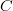$C$(i.e. oscillations with a wave-length equal to one or two widths of the horizontal resolution):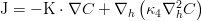$\mathrm{J} = -\mathrm{K}\cdot \nabla C + \nabla_{h}\left( \kappa_4 \nabla_{h}^2 C \right)$

where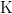$\mathrm{K}$ is Fick's law diffusivity. A ready made formula that would estimate the order of magnitude of the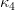$\kappa_4$ coefficient for momentum would be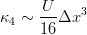$\kappa_4 \sim \frac{U}{16} \Delta x^3$

where U is a typical flow velocity and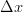$\Delta x$ is the numerical model horizontal resolution.

## MOHID

In mohid, you need to add in the Hydrodynamic_x.dat the following keywords:

BIHARMONIC        : [0/1] <-- Activates de biharmonic viscous flux
BIHARMONIC_COEF   : [Real] <-- Sets the value of$\kappa_4$